# Dynamic Graphics in R

## 1  Introduction

The tcltk package of the R programming language gives you simple access to the Tcl/Tk toolkit originally developed by John Ousterhout. This toolkit provides a variety of widgets, and has been ported to many computer platforms. Interfaces to the toolkit appear in a number of programming languages including perl and python, so learning some Tcl/Tk commands may prove useful in other settings as well as within R. Since R has its own plotting subsystem, there's no need to worry about actually creating graphics through Tcl/Tk; the toolkit is only used to produce controls, not to draw the actual graphs. There are two approaches to plotting with the tcltk package: the first uses R's usual graphics window, with any controls being displayed in a separate window, while the second displays your plot as an image in a window that can contain other graphical elements.
The remainder of this paper will present some basic concepts which you may find useful when getting started with the tcltk package. The final section contains a complete example of a function that allows you to dynamically vary the span of the super smoother algorithm, and to display that algorithm's default smooth.

## 2  Some Tk basics

The various controls that might appear as part of a dynamic graphics display are known collectively as "widgets". Some examples of widgets are radiobuttons, scales, simple pushbuttons, listboxes, etc. The basic widget in Tk is the frame, which is essentially a container for any other widgets you wish to use. You can have as many frames as you want, but each individual entity which appears on the screen must be in a "top level" frame, created with the tktoplevel function. When using Tk, you first create a widget (specifying its appearance, the command to be executed when the widget is activated, etc.), and then you specify how it should appear in its parent frame. While Tk provides a number of different schemes for achieving this, we'll discuss the technique known as "packing". The basic idea is to group together widgets (and accompanying labels, if appropriate) into a series of frames, and then to call the tkpack function to put the widgets in the frames, then to finally pack the frames into a main frame which is contained in a top-level widget. The tkpack function takes the parent frame as its first argument and the frame to be packed as the second argument. By default, objects are backed into a frame from top to bottom, with each object being centered within its parent frame. You can control how widgets get packed into a frame by the order in which you call tkpack, and the optional side= argument which can take the values "left", "right", "top" or "bottom". To align packed widgets, you can specify an anchor= argument, which can take values representing compass directions like "n", "e", "sw", etc.
When a button is pressed or a slider is moved, you may want to call a function as a result of the event. Such functions are know as callbacks. To specify a callback, you pass a function as the command= argument when first creating the widget. For simple tasks (like a quit button), you can use an anonymous function, but one useful strategy in preparing dynamic graphics using the tcltk package is to write a set of functions which you want controlled by your widgets, and simply use those function names as the command= argument. Functions created for this do not accept arguments, so you may need to use frame 0 variables, i.e. variables set using the <<- operator in place of <-, to keep track of information. In addition, it may be necessary to use "..." as the argument list of your callback functions.
In addition, there are a wide range of events (key presses, mouse clicks, hitting return, moving the mouse in or out of a widget's space, etc.) which can be associated with commands of your choice. The process of associating an event with an action is known as binding, and is implemented in R through the tkbind function, providing three arguments: the widget, the event, and the action to be performed. Events are specified as a character variable with the event's name surrounded by angle brackets. A small sampling of events is listed below; refer to a manual on the X window system or Tk for more information. (In the list that follows, the letter "x" is used to represent any key on the keyboard.)
```<Return>                  <FocusIn>
<Key-x>                   <FocusOut>
<Alt-x>                   <Button1>, <Button2>, etc.
<Control-x>               <ButtonRelease1>, <ButtonRelease2>, etc.
<Destroy>                 <Double-Button1>, <Double-Button2>, etc.
<Triple-Button1>, <Triple-Button2>, etc.

```
Finally, to change properties of widgets after they are created, you can use the tkconfig function. You call this function with the widget whose properties you wish to change, followed by a comma-separated list of named arguments corresponding to the properties you wish to change. In practice, you need to follow a call to tkconfig with a call to tcl('update') in order to actually see the changes.

## 3  Some Common Widgets

Note:  Each of the widget functions takes, as its first argument, the parent frame into which it will eventually be packed. In addition, most widgets accept height= and width= arguments to specify their size, but, when using the tkpack function, there's usually no need to provide this information. Any arguments mentioned in the following subsections appear after the parent frame.

### 3.1  Frames: tkframe

The tkframe function creates a frame, which serves as the building block of all Tk applications. You can modify the frame's appearance through arguments such as borderwidth, background (to specify background color), and relief (which takes a value from among "groove", "flat", "raised", "ridge", "solid", or "sunken").

### 3.2  Labels: tklabel

The tklabel function is used to produce a simple text label. By packing a label into a frame before packing some other widget, you can put an identifying label on a widget. In addition to the argument text, which specifies the text to appear in the label, you can specify font, and size to control the appearance of the text, and, background and foreground to control the color of the label.

### 3.3  Buttons: tkbutton

To create a pushbutton which, when activated, will carry out some command, use the tkbutton function. This function accepts an argument text, specifying the text to appear on the button, as well as command, which is a function that will be executed when the button is pressed.

### 3.4  Sliders: tkscale

You can create a slider to change the value of a variable using the tkscale function. Among its arguments are from, to and resolution, which control the values which will be set; showvalue, which, if set to TRUE will display the value of the variable above the slider, and orient which takes values "horizontal" or "vertical". You specify the name of a Tcl variable to be controlled by the slider through the argument variable, and a function to be called each time the variable's value changes through the argument command. Note that the variable controlled by the slider is a Tcl variable, not an R variable. This means that you must use the tclvar function to create the variable (passing an optional initialization value if desired), and the tclvalue function to access its value.

To provide a set of choices where only one choice can be active at any given time, use the tkradiobutton function. Each radio button is created and packed separately, but should specify the same variable= argument (similar to the variable argument to tkscale) as other radiobuttons in the same group. The value argument to tkradiobutton provides the value that the specified variable should take when the particular button is selected. If a command argument is specified, it will be executed any time the button is selected; without such an argument, you would need at least one widget somewhere in your application that specified some command to be executed.

### 3.6  Checkbuttons: tkcheckbutton

Checkbuttons are like radio buttons, but more than one choice among a set of buttons is allowable. Arguments are similar to those of tkradiobutton, but you need to specify a separate Tcl variable for each checkbutton you produce. Since these names are character strings, they can be generated very easily using the paste function. However, the tclvar variable mentioned previously is a psuedo-list, and does not support subscripting. Suppose you wish to access a Tcl variable whose name in stored in a variable called varname. (Note that the Tcl variable's name is not varname; it's name is simply stored in that variable.) You would need to evaluate an expression like eval(parse(text=paste("tclvar\$",varname,sep="")) in order to retrieve the value of the variable.

### 3.7  Text Entry: tkentry

To allow for the entry of arbitrary text, use the tkentry function. The width argument specifies the width of the entry field, and the textvariable argument specifies the name of a Tcl variable to contain the entered text.

## 4  Example 1: Interaction Counter

This very simple example creates a frame with a label, and updates the label each time an iteration of a loop is carried out.
```require(tcltk) || stop("tcltk support is absent")
base <- tktoplevel()
tkwm.title(base,'Label Test')
frm <- tkframe(base)

lab <- tklabel(frm,text="  ",width=20)
tkpack(frm,lab)

```
```res <- matrix(0,10,10)
for(i in 1:10){
tkconfigure(lab,text=paste(i))
tcl('update')
for(j in 1:10){
z <- rnorm(15000)
res[i,j] <- mean(z)
}
}

```

## 5  Example 2: Histogram Maker

This example produces a frame with a text entry field to allow entering a data frame name. When the return key is hit inside the entry field, the frame expands to include checkbuttons for each variable, a button to create the histograms, and another button to quit. The appearance of the frame, both before and after hitting return inside the text entry area, is shown in Figure 1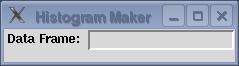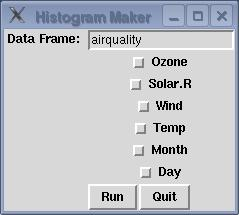Figure 1: Histogram maker
```  require(tcltk) || stop("tcltk support is absent")

makebuttons <- function(...){
df <- get(tclvalue(dataframe))
varfr <- tkframe(infrm)
k <- 1
for (n in names(df)){
assign(paste("X",k,sep=""),tclVar(""),pos=1)
tkpack(varfr,tkcheckbutton(varfr,text=n,variable=get(paste("X",k,sep="")),anchor='w'))
k <- k + 1
}
tkpack(varfr,infrm)

bfrm <- tkframe(infrm)
tkpack(bfrm,tkbutton(bfrm,text='Run',command=doit),side='left')
tkpack(bfrm,tkbutton(bfrm,text='Quit',command=function()tkdestroy(base)))
tkpack(bfrm,infrm)

}

doit <- function(...){
df <- get(tclvalue(dataframe))
use <- NULL
for(i in 1:dim(df)[]){
thevar <- get(paste("X",i,sep=""))
check <- tclvalue(thevar)
if(check == '1')use <- c(use,i)
}
for(i in use){
hist(df[,i],main=names(df)[i])
}
}

base <- tktoplevel()
tkwm.title(base,'Histogram Maker')
frm <- tkframe(base)

infrm <- tkframe(frm)
tkpack(infrm,tklabel(infrm,text="Data Frame: "),side='left',anchor='n')
dataframe <- tclVar("")
dfentry <- tkentry(infrm,width=20,textvariable=dataframe)
tkbind(dfentry,"<Return>",makebuttons)
tkbind(dfentry,"<Destroy>",function()print('Hello world'))
tkpack(infrm,dfentry)

tkpack(frm,infrm)

```

## 6  Example 3: Slider Control for Supersmoother

This example consists of a function which creates a slider to change the smoothing parameter sent to the R function supersmu. In addition, a button is provided to display supersmu's default smoothing of the data as a dotted line. Note the order in which graphing commands are executed: by calling plot with arguments which don't vary, we make sure that the axes of the graph don't change. The appearance of the frame containing the slider and the buttons is shown in Figure 2.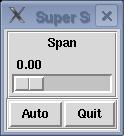Figure 2: Supersmoother Control
```supsmufn <- function(x,y){

library(modreg)
require(tcltk) || stop("tcltk support is absent")
supsmufn.auto <<- 0

chngplt <- function(...){
span <- as.numeric(tclvalue(span))
if(span == 0)span <- 1e-6
smth <- supsmu(x,y,span=span)
plot(x,y)
lines(smth\$x,smth\$y)
if(supsmufn.auto == 1)dobaseplot()

}

baseplot <- function(...){
if(supsmufn.auto == 0)supsmufn.auto <<- 1
else supsmufn.auto <<- 0

if(supsmufn.auto)dobaseplot(x,y)
}

dobaseplot <- function(...){
smth <- supsmu(x,y)
lines(smth\$x,smth\$y,lty=2)
}

base <- tktoplevel()
tkwm.title(base,"Super Smoother")

mainfrm <- tkframe(base,borderwidth=2)

slide <- tkframe(mainfrm,relief="groove",borderwidth=2)
tkpack(tklabel(slide,text="Span"))

span <- tclVar(0)
tkpack(tkscale(slide,command=chngplt,from=0,to=1.0,showvalue=T,
variable=span,resolution=.01,orient="horiz"))
tkpack(mainfrm,slide)

butfrm <- tkframe(mainfrm,borderwidth=2,relief="groove")
a.but <- tkbutton(butfrm,command=baseplot,text="Auto")
q.but <- tkbutton(butfrm,text="Quit",command=function()tkdestroy(base))
tkpack(butfrm,a.but,side="left")
tkpack(butfrm,q.but,side="right")
tkpack(mainfrm,butfrm)
}

```

## 7  Using tkrplot for plotting

As mentioned in the introduction, a second method for plotting allows placing controls in the same window as the plot itself. The tkrplot package provides the necessary functions to create an image representing your plot, and to automatically redraw it when necessary.
To use the tkrplot library, a tkrplot widget is created using the tkrplot function: this function accepts two arguments: the frame in which the plot is to be displayed, and the name of a function (with ... as its only argument) which will do the actual plotting.
To illustrate, consider a GUI consisting of a plotting window displaying the smoothed density of a vector of data, with a slider directly below the plot window to control the smoothing bandwidth. First, we create a toplevel window, a variable to hold the width, and write a function that will create the appropriate display:
```tt = tktoplevel()
width = tclVar(.5)
plotdens = function(...){
wid = as.numeric(tclvalue(width))
plot(density(x,bw=wid))
}

```
Next, we create the tkrplot widget using this function, and create a trivial updating function that simply calls tkrreplot:
```img = tkrplot(tt,plotdens)
densplot = function(...)tkrreplot(img)

```
Finally we create a slider to call the updating function when its value is changed, and pack all the widgets:
```scl = tkscale(tt,command=densplot,from=0.1,to=2,showvalue=TRUE,
variable=width,resolution=0.1,orient='horiz')
tkpack(img,side='top')
tkpack(scl,side='top')

```
The GUI is illustrated below using a mixture of normal distributions, and two different bandwidths.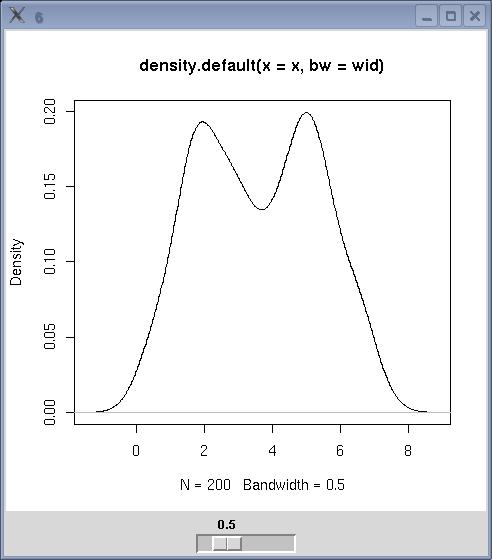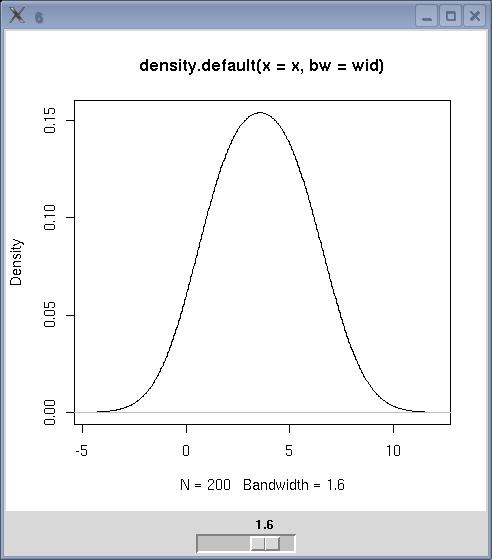Figure 3: Density Smoother

File translated from TEX by TTH, version 3.67.
On 5 Mar 2008, 09:04.